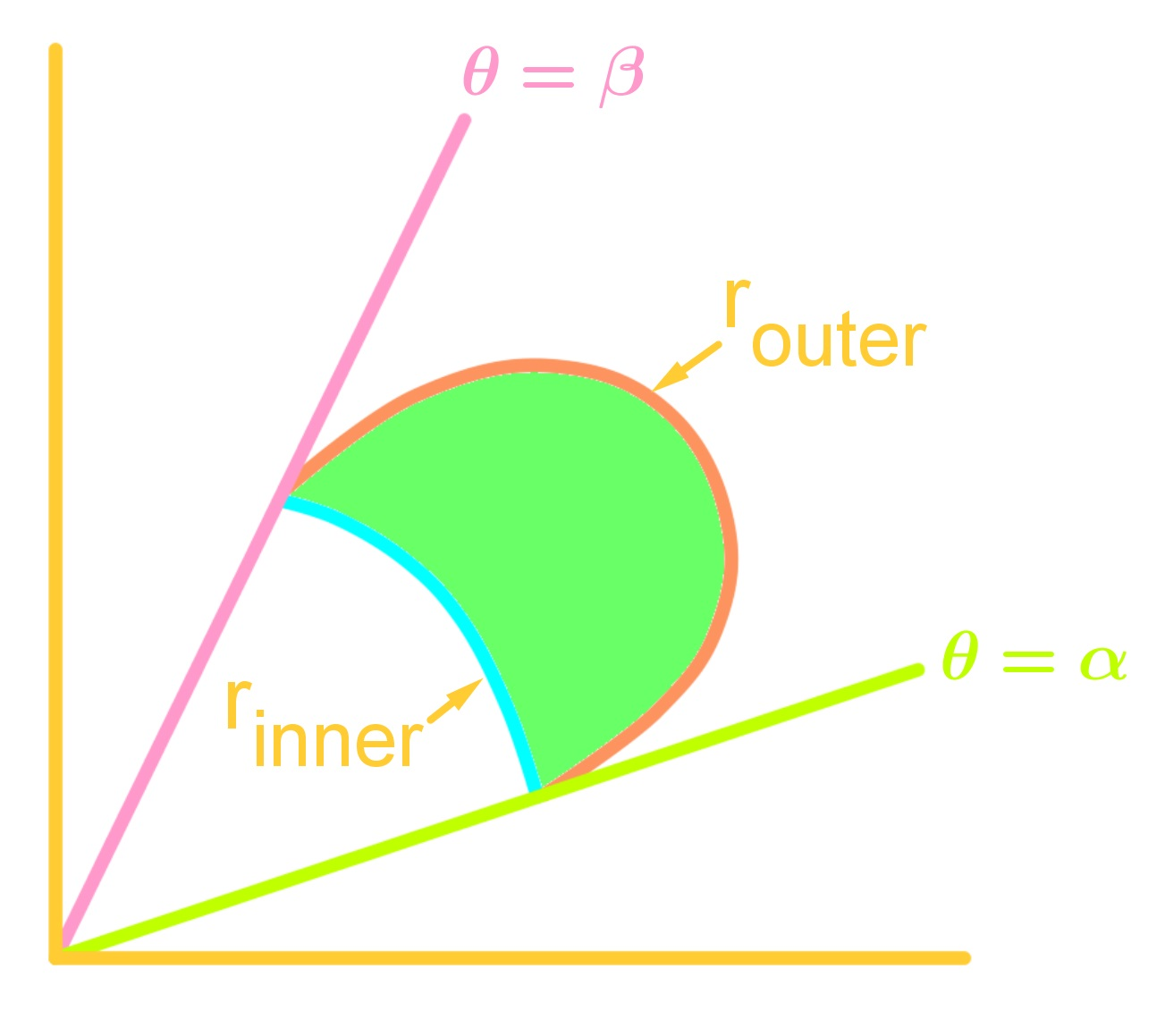# Area of polar curves - Parametric Equations and Polar Coordinates

### Area of polar curves

In this section, we will learn how to find the area of polar curves. For polar curves, we do not really find the area under the curve, but rather the area of where the angle covers in the curve. Note that not only can we find the area of one polar equation, but we can also find the area between two polar equations. It is important to always draw the curves out so that you can locate the area you are integrating, and write the integral correctly. We will first examine a generalized formula in finding areas of polar curves. Then we will apply this to finding areas with one polar curve and then two polar curves. Make sure you know your trigonometric identities very well before tackling these questions.

#### Lessons

###### To find the area of a single polar equation, we use the following formula: $A=\int_{\alpha}^{\beta}\frac{1}{2}r^2d\theta$ where $\alpha$ is the starting angle and $\beta$ is the ending angle. To find the area that is enclosed by two polar equations like in the picture below, we use the formula: $A=\int_{\alpha}^{\beta}\frac{1}{2}(r^{2}\;_{outer}-r^{2}\;_{inner})d\theta$ where $r_{outer}$ is the outer part of the first polar equation, and $r_{inner}$ is the inner part of the second polar equation.